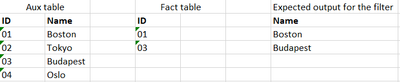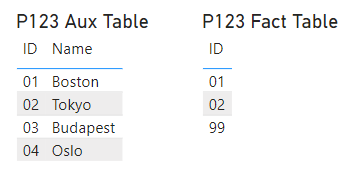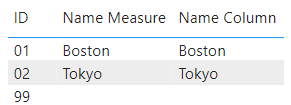cancel
Showing results for
Did you mean:Helper III

## To filter aux table values with the fact table values

I have a request that to show only the values in the filter which can be found in my fact table, in a nutshell:My problem is that I can't use bi-directional relationships between the tables (in other words: I can't connect them on the modelling pane).

So I would like to create a DAX measure with USERELATIONSHIP which will return for my slicer the values which can be found in the fact table, but "translated" it with the aux table's Name field.

1 ACCEPTED SOLUTIONSuper User

I am not sure userelationship works in your way of explanation. Assuming you dont want to create relationships and want the text value from another table.

note: Relationships - active, inactive are always preferred approach.

Calculated column approach:

``Name Column = LOOKUPVALUE(P123_AuxTable[Name], P123_AuxTable[ID], P123_FactTable[ID])``

Measure approach:

``````Name Measure =
var _selVal = SELECTEDVALUE(P123_FactTable[ID])
var _name = calculate(Max(P123_AuxTable[Name]), P123_AuxTable[ID] = TREATAS( { _selVal }, P123_AuxTable[ID]))

return _name  ``````Data:

FYI: I added ID "99" to check the outlier.Output:Hope this helps!Super User

I am not sure userelationship works in your way of explanation. Assuming you dont want to create relationships and want the text value from another table.

note: Relationships - active, inactive are always preferred approach.

Calculated column approach:

``Name Column = LOOKUPVALUE(P123_AuxTable[Name], P123_AuxTable[ID], P123_FactTable[ID])``

Measure approach:

``````Name Measure =
var _selVal = SELECTEDVALUE(P123_FactTable[ID])
var _name = calculate(Max(P123_AuxTable[Name]), P123_AuxTable[ID] = TREATAS( { _selVal }, P123_AuxTable[ID]))

return _name  ``````Data:

FYI: I added ID "99" to check the outlier.Output:Hope this helps!